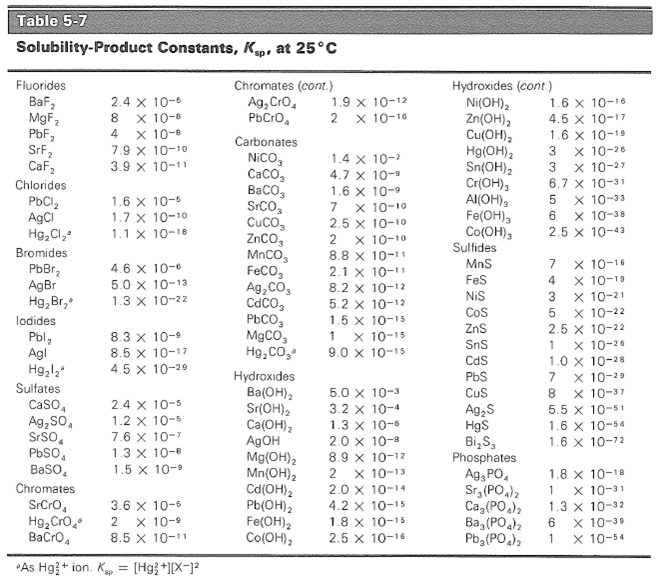## Solubility Product

#### Learning Objective

• Calculate the solubility product constant of an aqueous solution.

#### Key Points

• The general form of the solubility product constant (Ksp) for the equation: $aA (s) \rightleftharpoons bB (aq) + cC (aq)$ is $K_{sp} = [B]^b[C]^c$ .
• The smaller the solubility product, the lower the solubility. Solubility products are useful in predicting whether a precipitate will form under specified conditions.
• In an ICE table, the solubility of the solid is equal to the change (x) in the equilibrium calculation.

#### Terms

• solubilityThe amount of a substance that will dissolve in a given amount of solvent to give a saturated solution under specified conditions.
• precipitateA solid that exits the liquid phase of a solution.

The solubility product constant (Ksp) is the equilibrium constant for a solid that dissolves in an aqueous solution. All of the rules for determining equilibrium constants continue to apply. An equilibrium constant is the ratio of the concentration of the products of a reaction divided by the concentration of the reactants once the reaction has reached equilibrium. Consider this reaction:

$AgCl (s) \rightarrow Ag^+ (aq) + Cl^- (aq)$

The equilibrium expression for the reaction is:

$K_{eq} = \frac{[Ag^+][Cl^-]}{[AgCl]}$

Because the AgCl is a solid, its concentration before and after the reaction is the same. The equilibrium equation can therefore be rearranged as:

$K_{sp} = [Ag^+][Cl^-]$

For substances in which the ions are not in a 1:1 ratio, the stoichiometric coefficients of the reaction become the exponents for the ions in the solubility-product expression:

$PbCl_2 \rightleftharpoons Pb^{2+} + 2Cl^- \text{ gives }\ K_{sp} = [Pb^{2+}][Cl^-]^2$

$Ba_3(PO_4)_2 \rightleftharpoons 3Ba^{2+} + {2PO_4}^{2-} \text{ gives }\ K_{sp}=[Ba^{2+}]^3[{PO_4}^{2-}]^2$

## Calculating the Solubility Product

At a certain temperature, the solubility of Fe(OH)2 in water is 7.7 x 10-6 mol/L (M).

Its Ksp can be calculated based on the equilibrium equation:

$Fe(OH)_2 \rightleftharpoons Fe^{2+} + 2OH^-$

Therefore, the solubility product expression is:

$K_{sp} = [Fe^{2+}][OH^-]^2$

One mole of dissolved Fe(OH)2 produces one mole of Fe2+ and two moles of OH, so therefore:ICE table for the solubility of Fe(OH)2The solubility of Fe(OH)2 is 7.7 x 10-6 M, this is equal to the value of the change (x) in the table.

$K_{sp} = x(2x)^2$ where x = 7.7 x 10-6

$[Fe^{2+}] = 7.7 \times 10^{-6}$

$[OH^-] = 2 \times 7.7 \times 10^{-6} = 1.54 \times 10^{-5}$

$K_{sp} = [Fe^{2+}][OH^-]^2$

$K_{sp} = 7.7\times10^{-6} \times (1.54\times10^{-5})^2$

$K_{sp} = 1.83\times10^{-15}$

## Uses of Solubility Product

Solubility products are useful in predicting whether a precipitate will form under specified conditions. It is also helpful in choosing conditions under which two chemical substances in solution can be separated by fractional precipitation. The solubility product of a number of substances have been experimentally determined and can be used to predict solubility at a specified temperature.Solubility product constants of common ionsThe solubility product constants of a number of substances. Substances are grouped by anion and listed in the order of decreasing Ksp; anions are listed roughly in order of decreasing solubility.